## Summary

Another form of noise is voronoi noise. For voronoi noise we need a bunch of points, then we generate a pattern based on which point is the closest. This specific implementation of voronoi noise will work based on cells just like most of the previous noise types we explored, this makes it relatively cheap and easy to repeat. To understand this tutorial I recommend you to have at least understood the basics of shaders in unity and how to generate random values in shaders.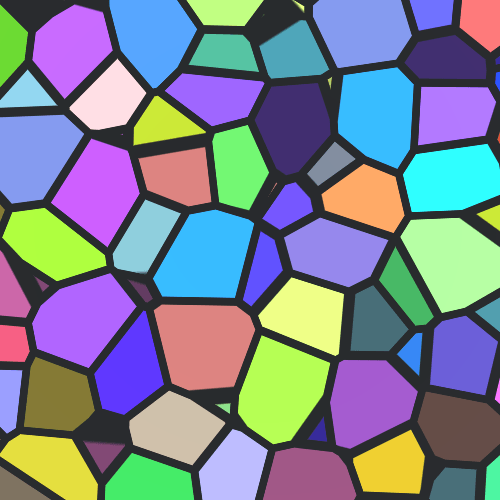Also possible trypophobia warning for this tutorial, visualising distances can look a bit messy.

## Get Cell Values

For our implementation of voronoi noise each of our cells will have one point. We start by implementing that in 2d. We start by simply dividing our space into cells by flooring the input value and generating random positions inside of the cells based on that. Then we calculate the distance to the input value based on that and return the distance. Just like in the previous noise tutorials we’ll base the noise on the world position so we don’t have to worry about scaling and uv mapping. We’ll also make the cell size adjustable by dividing the value by a cell size property before passing it to the noise function.

``````Shader "Tutorial/028_voronoi_noise/2d" {
Properties {
_CellSize ("Cell Size", Range(0, 2)) = 2
}
Tags{ "RenderType"="Opaque" "Queue"="Geometry"}

CGPROGRAM

#pragma target 3.0

#include "Random.cginc"

float _CellSize;

struct Input {
float3 worldPos;
};

float voronoiNoise(float2 value){
float2 cell = floor(value);
float2 cellPosition = cell + rand2dTo2d(cell);
float2 toCell = cellPosition - value;
float distToCell = length(toCell);
return distToCell;
}

void surf (Input i, inout SurfaceOutputStandard o) {
float2 value = i.worldPos.xz / _CellSize;
float noise = voronoiNoise(value);

o.Albedo = noise;
}
ENDCG
}
FallBack "Standard"
}
``````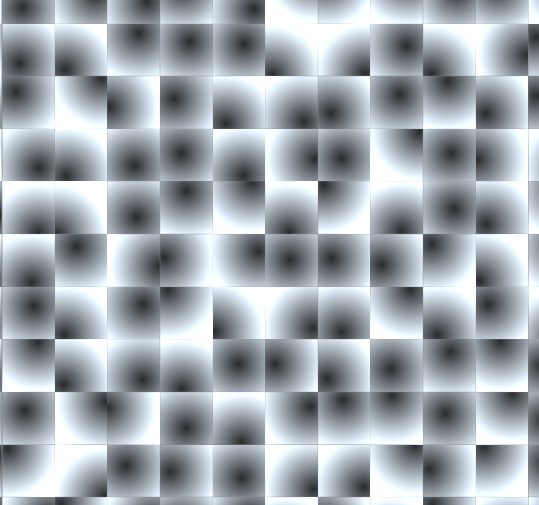But we need more than just the center of the cell we’re in ourselves. We also have to sample the neighboring cells to see which cell center is actually the closest. For that we use for loops which go from -1 to +1. In each iteration we check if the distance to the cell we’re checking is smaller than the previous closest cell position, if it is, we replace the distance. The variable we write the distance to has to be declared outside of the shader and has to have a default value that’s bigger than any distance between 2 points in the 3x3 grid we check. We tell the compiler to unroll the loops to get better performance in the shader.

``````float voronoiNoise(float2 value){
float2 baseCell = floor(value);

float minDistToCell = 10;
[unroll]
for(int x=-1; x<=1; x++){
[unroll]
for(int y=-1; y<=1; y++){
float2 cell = baseCell + float2(x, y);
float2 cellPosition = cell + rand2dTo2d(cell);
float2 toCell = cellPosition - value;
float distToCell = length(toCell);
if(distToCell < minDistToCell){
minDistToCell = distToCell;
}
}
}
return minDistToCell;
}
``````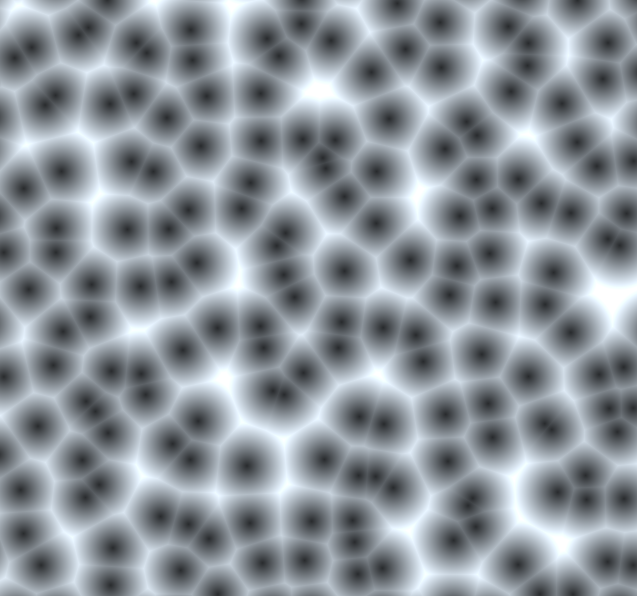But we usually don’t just want the distance to the nearest point, we also want to know which point that is. To get that we simply add a new value which we also write to in the if statement. In it we save the position of the nearest cell. After we have the nearest cell we can generate a identifier based on it with the random function and return it. We can return the distance to the cell position as well as the random value if we simply change the function to return a 2d vector. We then return the distance to the position as the x component and the random value as the y component. In the surface function we then simply use the y component of the return value.

``````float voronoiNoise(float2 value){
float2 baseCell = floor(value);

float minDistToCell = 10;
float2 closestCell;
[unroll]
for(int x=-1; x<=1; x++){
[unroll]
for(int y=-1; y<=1; y++){
float2 cell = baseCell + float2(x, y);
float2 cellPosition = cell + rand2dTo2d(cell);
float2 toCell = cellPosition - value;
float distToCell = length(toCell);
if(distToCell < minDistToCell){
minDistToCell = distToCell;
closestCell = cell;
}
}
}
float random = rand2dTo1d(closestCell);
return float2(minDistToCell, random);
}
``````
``````void surf (Input i, inout SurfaceOutputStandard o) {
float2 value = i.worldPos.xz / _CellSize;
float noise = voronoiNoise(value).y;
o.Albedo = noise;
}
``````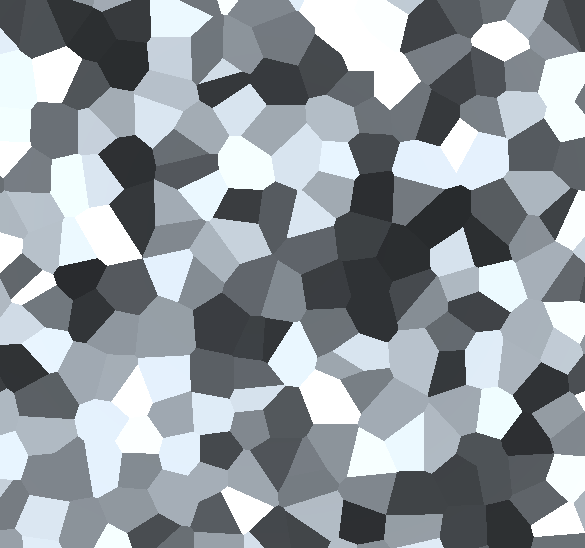Because it’s the same value in the same cell we can then use this identifier in the surface function to generate more colorful values if we want to. To do that we simply feed the return value into the 1dTo3d random function.

``````void surf (Input i, inout SurfaceOutputStandard o) {
float2 value = i.worldPos.xz / _CellSize;
float noise = voronoiNoise(value).y;
float3 color = rand1dTo3d(noise);
o.Albedo = color;
}
``````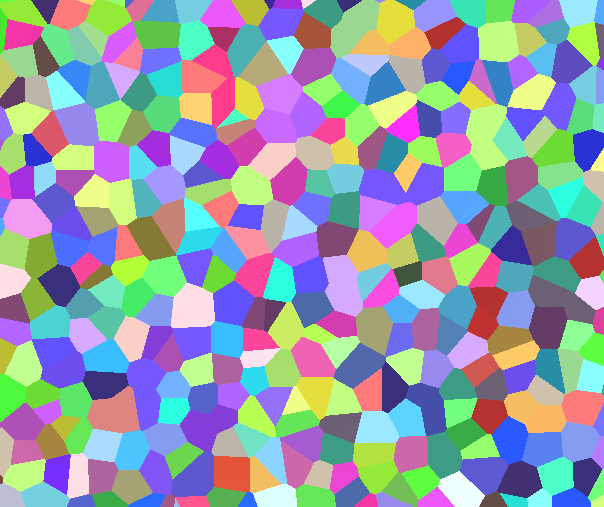## Getting the distance to the border

We already have the distance to the center of the cell, but for many effects, like drawing edges between the cells, we also want the distance to the border. A common way to calculate that is to calculate both the nearest and the second nearest point and then subtract the distance to the nearest point from the distance to the second nearest point. This technique is fast, but doesn’t deliver very accurate results. The method we’re using instead will calculate the distance to every edge and take the shortest distance.

To calculate the distance we iterate through the neighboring cells again, this time with the information which cell is the closest. We then calculate the distance to the border by first calculating the center between the two cell positions and then the vector from the sample point to this center. Once we have that, we also calculate the vector from the closest cell to the cell we’re calculating the distance to the border to. We then also normalize that difference vector so it has a length of one.

After we calculated those vectors, we can then calculate the dot product between the cell difference and the vector to the center between the cells. The dot product tells us how far the vector to the center is in the direction and in relation to the vector between the cells. So because we normalized the difference vector it tells us the distance in units (not unity units though, because we changed the value size before passing it to the method by dividing the cell size).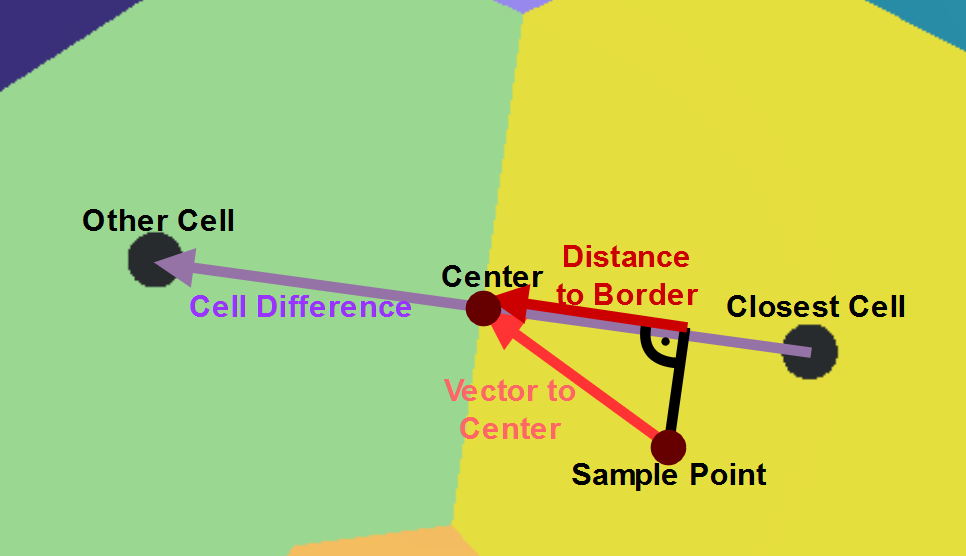For the implementation we also remember the vector to the closest cell in our first pass so we can use it for the calculation later. Then for the second pass we create a new variable to hold the distance to the closest edge. The iterations looks just like in the first pass, two loops from -1 to +1. We’ll change the names of the iteration variables in the first loops to x1 and y1 and in the second to x2 and y2, otherwise with unrolling the loops the shader compiler can get confused and give us warnings.

Then in the inner loop we also calculate the cell, the position of the cell and the vector from the sample point to the cell just like in the first pass. Then the part where we actually calculate the distance to the border will be in a if statement. That’s because if we calculate the distance to the border between the nearest cell and itself, that will always tell us the border is 0 units away, which is closer than the borders we actually care about. To check if the cell is the closest cell, we subtract the closest cell from the cell we’re checking at the moment to get the difference to the closest cell. Then we take the absolute value of that and add the x and y components. Then we can check if that sum is lower than some threshold and if it is we know that we’re in the closest cell and just don’t do the comparison with the edge. The reason why we can’t just use `==` to check if the cells are equal is that we’re working with floating point numbers because shaders love floating point numbers, but they’re often not exactly the same.

Then in the if statement which checks that we’re not in the closest cell, we do the distance calculation. First we calculate the vector from the sample point to the center between the cell points. Since we already have the vectors to the cell we’re checking and to the closest cell we can simply take their average by adding them together and dividing the sum by 2. The next variable we need is the normalised difference between the two cells, to get that we simply subtract the vector to the closest cell from the vector to the cell we’re checking and then normalise the result.

The last step to get the distance to the edge is then simply take the dotproduct between the vector to the center and the vector between the cell positions. After getting that successfully we then set the minimum edge distance to the minimum of the distance so far and the distance to the new edge. We also could’ve use the minimum in the first loop to just get the distance to the closest cell and it would’ve been faster, but the if statement allows us to save more information.

After calculating the distance to the closest edge we can then expand the output vector to a vector 3 and write the distance to the edge in the z component.

``````float3 voronoiNoise(float2 value){
float2 baseCell = floor(value);

//first pass to find the closest cell
float minDistToCell = 10;
float2 toClosestCell;
float2 closestCell;
[unroll]
for(int x1=-1; x1<=1; x1++){
[unroll]
for(int y1=-1; y1<=1; y1++){
float2 cell = baseCell + float2(x1, y1);
float2 cellPosition = cell + rand2dTo2d(cell);
float2 toCell = cellPosition - value;
float distToCell = length(toCell);
if(distToCell < minDistToCell){
minDistToCell = distToCell;
closestCell = cell;
toClosestCell = toCell;
}
}
}

//second pass to find the distance to the closest edge
float minEdgeDistance = 10;
[unroll]
for(int x2=-1; x2<=1; x2++){
[unroll]
for(int y2=-1; y2<=1; y2++){
float2 cell = baseCell + float2(x2, y2);
float2 cellPosition = cell + rand2dTo2d(cell);
float2 toCell = cellPosition - value;

float2 diffToClosestCell = abs(closestCell - cell);
bool isClosestCell = diffToClosestCell.x + diffToClosestCell.y < 0.1;
if(!isClosestCell){
float2 toCenter = (toClosestCell + toCell) * 0.5;
float2 cellDifference = normalize(toCell - toClosestCell);
float edgeDistance = dot(toCenter, cellDifference);
minEdgeDistance = min(minEdgeDistance, edgeDistance);
}
}
}

float random = rand2dTo1d(closestCell);
return float3(minDistToCell, random, minEdgeDistance);
}
``````
``````void surf (Input i, inout SurfaceOutputStandard o) {
float2 value = i.worldPos.xz / _CellSize;
float3 noise = implVoronoiNoise(value);
o.Albedo = noise.z;
}
``````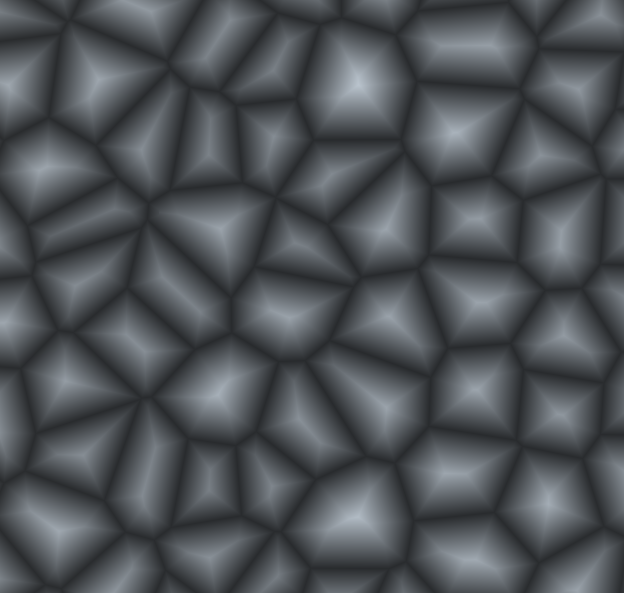## Visualising voronoi noise

Now we have the 3 variables based on the points. The distance to the point, a random value generated based on the point and the distance to the border to the nearest cell. We already showed how to generate more interresting colors based on the random value. Another thing that’s often used is to draw the borders based on the distance to the nearest border. For that we first decide whats a border and what’s not. We can do that via the step function, we pass it two values and it’ll return 1 if the first argument is greater or equal than the second and 0 if the second one is greater. After we decided what’s a border and what isn’t, we can interpolate from the color of the cell to a borderColor based on that variable. We’ll add the border color as a property so we can change it from the inspector.

``````void surf (Input i, inout SurfaceOutputStandard o) {
float2 value = i.worldPos.xz / _CellSize;
float3 noise = voronoiNoise(value);

float3 cellColor = rand1dTo3d(noise.y);
float isBorder = step(noise.z, 0.05);
float3 color = lerp(cellColor, _BorderColor, isBorder);
o.Albedo = color;
}
``````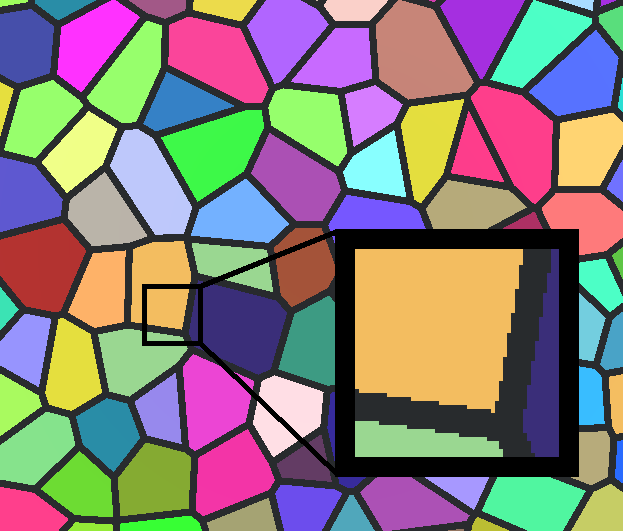One little issue of drawing the borders like this is that border or no border is a binary choice, it’s either one of the other. This leads to so aliasing artefacts where the pixels of the rendered image are very obvious. With a simple trick we can blur the line though. We simply get how much the border distance changes in the neighboring pixels and and then do a interpolation based on that, the cutoff value minus the change in value is 0, the cutoff value plus the change in distance is 1 and all values inbetween are interpolated inbetween.

Because we defined the cell border distance to be in the same scale as the input value we can get the best results by evaluating how much the input changes in the neighboring pixels. We first get the change of the input by passing the variable to `fwidth`, that function will then return us the change over the neighboring pixels. But because the value is a 2d value, the return value is also 2d, to get the scalar length we need, we simply calculate the length of the difference. Because we use the change value both in the positive and negative direction, we then also half it, otherwise the result can look too blurry (I encourage you to change the multiplier of the change result here and how it looks at different distances).

After we calculate the value change we can then use it to blur the edges. We replace the `step` function with the `smoothstep` function which will allow us to pass 2 values and it’ll return a value between 0 and 1 just like described earlier. It’s important for us to also calculate 1 minus the result afterwards, because the smoothstep will return 0 if it’s a a border and 1 when it isn’t and we want our varaiable to indicate if it’s a border.

Because we used a linear interpolation to decide between the border and the cell color we don’t have to change anything else to make this work, the values between border and not border automatically correspond to the colors between the border color and the cell color.

``````void surf (Input i, inout SurfaceOutputStandard o) {
float2 value = i.worldPos.xz / _CellSize;
float3 noise = voronoiNoise(value);

float3 cellColor = rand1dTo3d(noise.y);
float valueChange = length(fwidth(value)) * 0.5;
float isBorder = 1 - smoothstep(0.05 - valueChange, 0.05 + valueChange, noise.z);
float3 color = lerp(cellColor, _BorderColor, isBorder);
o.Albedo = color;
}
``````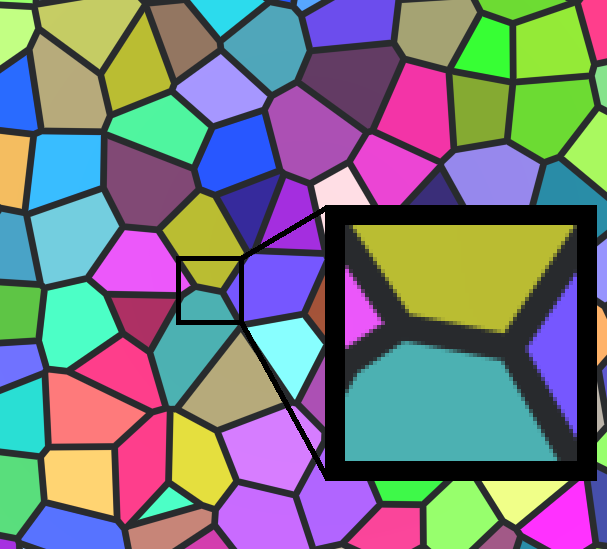## 3d Voronoi

To change the voronoi noise to more dimensions we change the input vector to a 3d vector. Then we also change all directions and cells that were previously 2d to 3d. Next we add another loop to both passes so we can check a 3x3x3 area of cells. We also factor the z component in when checking if the cell we’re modifying is the closest cell.

In the surface shader we change the value to use all directional axes before we pass it into the noise function. Another whing we’ll change is that we won’t base the valueChange for smoothing the borders from the input value anymore, instead we use the distance from the border directly. That’s because now the value isn’t 2d like the surface anymore and the borders can run in many angles to the surface which makes the previous way of getting the value result in way too smooth edges sometimes.

``````float3 voronoiNoise(float3 value){
float3 baseCell = floor(value);

//first pass to find the closest cell
float minDistToCell = 10;
float3 toClosestCell;
float3 closestCell;
[unroll]
for(int x1=-1; x1<=1; x1++){
[unroll]
for(int y1=-1; y1<=1; y1++){
[unroll]
for(int z1=-1; z1<=1; z1++){
float3 cell = baseCell + float3(x1, y1, z1);
float3 cellPosition = cell + rand3dTo3d(cell);
float3 toCell = cellPosition - value;
float distToCell = length(toCell);
if(distToCell < minDistToCell){
minDistToCell = distToCell;
closestCell = cell;
toClosestCell = toCell;
}
}
}
}

//second pass to find the distance to the closest edge
float minEdgeDistance = 10;
[unroll]
for(int x2=-1; x2<=1; x2++){
[unroll]
for(int y2=-1; y2<=1; y2++){
[unroll]
for(int z2=-1; z2<=1; z2++){
float3 cell = baseCell + float3(x2, y2, z2);
float3 cellPosition = cell + rand3dTo3d(cell);
float3 toCell = cellPosition - value;

float3 diffToClosestCell = abs(closestCell - cell);
bool isClosestCell = diffToClosestCell.x + diffToClosestCell.y + diffToClosestCell.z < 0.1;
if(!isClosestCell){
float3 toCenter = (toClosestCell + toCell) * 0.5;
float3 cellDifference = normalize(toCell - toClosestCell);
float edgeDistance = dot(toCenter, cellDifference);
minEdgeDistance = min(minEdgeDistance, edgeDistance);
}
}
}
}

float random = rand3dTo1d(closestCell);
return float3(minDistToCell, random, minEdgeDistance);
}
``````
``````void surf (Input i, inout SurfaceOutputStandard o) {
float3 value = i.worldPos.xyz / _CellSize;
float3 noise = voronoiNoise(value);

float3 cellColor = rand1dTo3d(noise.y);
float valueChange = fwidth(value.z) * 0.5;
float isBorder = 1 - smoothstep(0.05 - valueChange, 0.05 + valueChange, noise.z);
float3 color = lerp(cellColor, _BorderColor, isBorder);
o.Albedo = color;
}
``````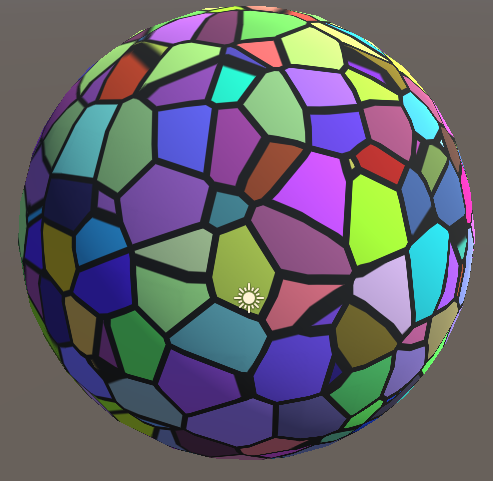## Scrolling noise

Just like the other kinds of noise we’re not limited to spacial dimensions here. We can use the the world dimensions for 2 axes and then animate the third based on the time. By going through the noise this way we can see the different cells begin and end.

``````void surf (Input i, inout SurfaceOutputStandard o) {
float3 value = i.worldPos.xyz / _CellSize;
value.y += _Time.y * _TimeScale;
float3 noise = voronoiNoise(value);

float3 cellColor = rand1dTo3d(noise.y);
float valueChange = fwidth(value.z) * 0.5;
float isBorder = 1 - smoothstep(0.05 - valueChange, 0.05 + valueChange, noise.z);
float3 color = lerp(cellColor, _BorderColor, isBorder);
o.Albedo = color;
}
``````## Source

### 2d Voronoi

``````Shader "Tutorial/028_voronoi_noise/2d" {
Properties {
_CellSize ("Cell Size", Range(0, 2)) = 2
_BorderColor ("Border Color", Color) = (0,0,0,1)
}
Tags{ "RenderType"="Opaque" "Queue"="Geometry"}

CGPROGRAM

#pragma target 3.0

#include "Random.cginc"

float _CellSize;
float3 _BorderColor;

struct Input {
float3 worldPos;
};

float3 voronoiNoise(float2 value){
float2 baseCell = floor(value);

//first pass to find the closest cell
float minDistToCell = 10;
float2 toClosestCell;
float2 closestCell;
[unroll]
for(int x1=-1; x1<=1; x1++){
[unroll]
for(int y1=-1; y1<=1; y1++){
float2 cell = baseCell + float2(x1, y1);
float2 cellPosition = cell + rand2dTo2d(cell);
float2 toCell = cellPosition - value;
float distToCell = length(toCell);
if(distToCell < minDistToCell){
minDistToCell = distToCell;
closestCell = cell;
toClosestCell = toCell;
}
}
}

//second pass to find the distance to the closest edge
float minEdgeDistance = 10;
[unroll]
for(int x2=-1; x2<=1; x2++){
[unroll]
for(int y2=-1; y2<=1; y2++){
float2 cell = baseCell + float2(x2, y2);
float2 cellPosition = cell + rand2dTo2d(cell);
float2 toCell = cellPosition - value;

float2 diffToClosestCell = abs(closestCell - cell);
bool isClosestCell = diffToClosestCell.x + diffToClosestCell.y < 0.1;
if(!isClosestCell){
float2 toCenter = (toClosestCell + toCell) * 0.5;
float2 cellDifference = normalize(toCell - toClosestCell);
float edgeDistance = dot(toCenter, cellDifference);
minEdgeDistance = min(minEdgeDistance, edgeDistance);
}
}
}

float random = rand2dTo1d(closestCell);
return float3(minDistToCell, random, minEdgeDistance);
}

void surf (Input i, inout SurfaceOutputStandard o) {
float2 value = i.worldPos.xz / _CellSize;
float3 noise = voronoiNoise(value);

float3 cellColor = rand1dTo3d(noise.y);
float valueChange = length(fwidth(value)) * 0.5;
float isBorder = 1 - smoothstep(0.05 - valueChange, 0.05 + valueChange, noise.z);
float3 color = lerp(cellColor, _BorderColor, isBorder);
o.Albedo = color;
}
ENDCG
}
FallBack "Standard"
}
``````

### 3d Voronoi

``````Shader "Tutorial/028_voronoi_noise/3d" {
Properties {
_CellSize ("Cell Size", Range(0, 2)) = 2
_BorderColor ("Border Color", Color) = (0,0,0,1)
}
Tags{ "RenderType"="Opaque" "Queue"="Geometry"}

CGPROGRAM

#pragma target 3.0

#include "Random.cginc"

float _CellSize;
float3 _BorderColor;

struct Input {
float3 worldPos;
};

float3 voronoiNoise(float3 value){
float3 baseCell = floor(value);

//first pass to find the closest cell
float minDistToCell = 10;
float3 toClosestCell;
float3 closestCell;
[unroll]
for(int x1=-1; x1<=1; x1++){
[unroll]
for(int y1=-1; y1<=1; y1++){
[unroll]
for(int z1=-1; z1<=1; z1++){
float3 cell = baseCell + float3(x1, y1, z1);
float3 cellPosition = cell + rand3dTo3d(cell);
float3 toCell = cellPosition - value;
float distToCell = length(toCell);
if(distToCell < minDistToCell){
minDistToCell = distToCell;
closestCell = cell;
toClosestCell = toCell;
}
}
}
}

//second pass to find the distance to the closest edge
float minEdgeDistance = 10;
[unroll]
for(int x2=-1; x2<=1; x2++){
[unroll]
for(int y2=-1; y2<=1; y2++){
[unroll]
for(int z2=-1; z2<=1; z2++){
float3 cell = baseCell + float3(x2, y2, z2);
float3 cellPosition = cell + rand3dTo3d(cell);
float3 toCell = cellPosition - value;

float3 diffToClosestCell = abs(closestCell - cell);
bool isClosestCell = diffToClosestCell.x + diffToClosestCell.y + diffToClosestCell.z < 0.1;
if(!isClosestCell){
float3 toCenter = (toClosestCell + toCell) * 0.5;
float3 cellDifference = normalize(toCell - toClosestCell);
float edgeDistance = dot(toCenter, cellDifference);
minEdgeDistance = min(minEdgeDistance, edgeDistance);
}
}
}
}

float random = rand3dTo1d(closestCell);
return float3(minDistToCell, random, minEdgeDistance);
}

void surf (Input i, inout SurfaceOutputStandard o) {
float3 value = i.worldPos.xyz / _CellSize;
float3 noise = voronoiNoise(value);

float3 cellColor = rand1dTo3d(noise.y);
float valueChange = fwidth(value.z) * 0.5;
float isBorder = 1 - smoothstep(0.05 - valueChange, 0.05 + valueChange, noise.z);
float3 color = lerp(cellColor, _BorderColor, isBorder);
o.Albedo = color;
}
ENDCG
}
FallBack "Standard"
}
``````

### Scrolling Voronoi

``````Shader "Tutorial/028_voronoi_noise/scrolling" {
Properties {
_CellSize ("Cell Size", Range(0, 2)) = 2
_BorderColor ("Border Color", Color) = (0,0,0,1)
_TimeScale ("Scrolling Speed", Range(0, 2)) = 1
}
Tags{ "RenderType"="Opaque" "Queue"="Geometry"}

CGPROGRAM

#pragma target 3.0

#include "Random.cginc"

float _CellSize;
float _TimeScale;
float3 _BorderColor;

struct Input {
float3 worldPos;
};

float3 voronoiNoise(float3 value){
float3 baseCell = floor(value);

//first pass to find the closest cell
float minDistToCell = 10;
float3 toClosestCell;
float3 closestCell;
[unroll]
for(int x1=-1; x1<=1; x1++){
[unroll]
for(int y1=-1; y1<=1; y1++){
[unroll]
for(int z1=-1; z1<=1; z1++){
float3 cell = baseCell + float3(x1, y1, z1);
float3 cellPosition = cell + rand3dTo3d(cell);
float3 toCell = cellPosition - value;
float distToCell = length(toCell);
if(distToCell < minDistToCell){
minDistToCell = distToCell;
closestCell = cell;
toClosestCell = toCell;
}
}
}
}

//second pass to find the distance to the closest edge
float minEdgeDistance = 10;
[unroll]
for(int x2=-1; x2<=1; x2++){
[unroll]
for(int y2=-1; y2<=1; y2++){
[unroll]
for(int z2=-1; z2<=1; z2++){
float3 cell = baseCell + float3(x2, y2, z2);
float3 cellPosition = cell + rand3dTo3d(cell);
float3 toCell = cellPosition - value;

float3 diffToClosestCell = abs(closestCell - cell);
bool isClosestCell = diffToClosestCell.x + diffToClosestCell.y + diffToClosestCell.z < 0.1;
if(!isClosestCell){
float3 toCenter = (toClosestCell + toCell) * 0.5;
float3 cellDifference = normalize(toCell - toClosestCell);
float edgeDistance = dot(toCenter, cellDifference);
minEdgeDistance = min(minEdgeDistance, edgeDistance);
}
}
}
}

float random = rand3dTo1d(closestCell);
return float3(minDistToCell, random, minEdgeDistance);
}

void surf (Input i, inout SurfaceOutputStandard o) {
float3 value = i.worldPos.xyz / _CellSize;
value.y += _Time.y * _TimeScale;
float3 noise = voronoiNoise(value);

float3 cellColor = rand1dTo3d(noise.y);
float valueChange = fwidth(value.z) * 0.5;
float isBorder = 1 - smoothstep(0.05 - valueChange, 0.05 + valueChange, noise.z);
float3 color = lerp(cellColor, _BorderColor, isBorder);
o.Albedo = color;
}
ENDCG
}
FallBack "Standard"
}
``````

I hope that I was able to explain voronoi noise clearly and that you’ll be able to create cool stuff with it.✨

You can also find me on twitter at @totallyRonja. If you liked my tutorial and want to support me you can do that on Patreon (patreon.com/RonjaTutorials) or Ko-Fi (ko-fi.com/RonjaTutorials).